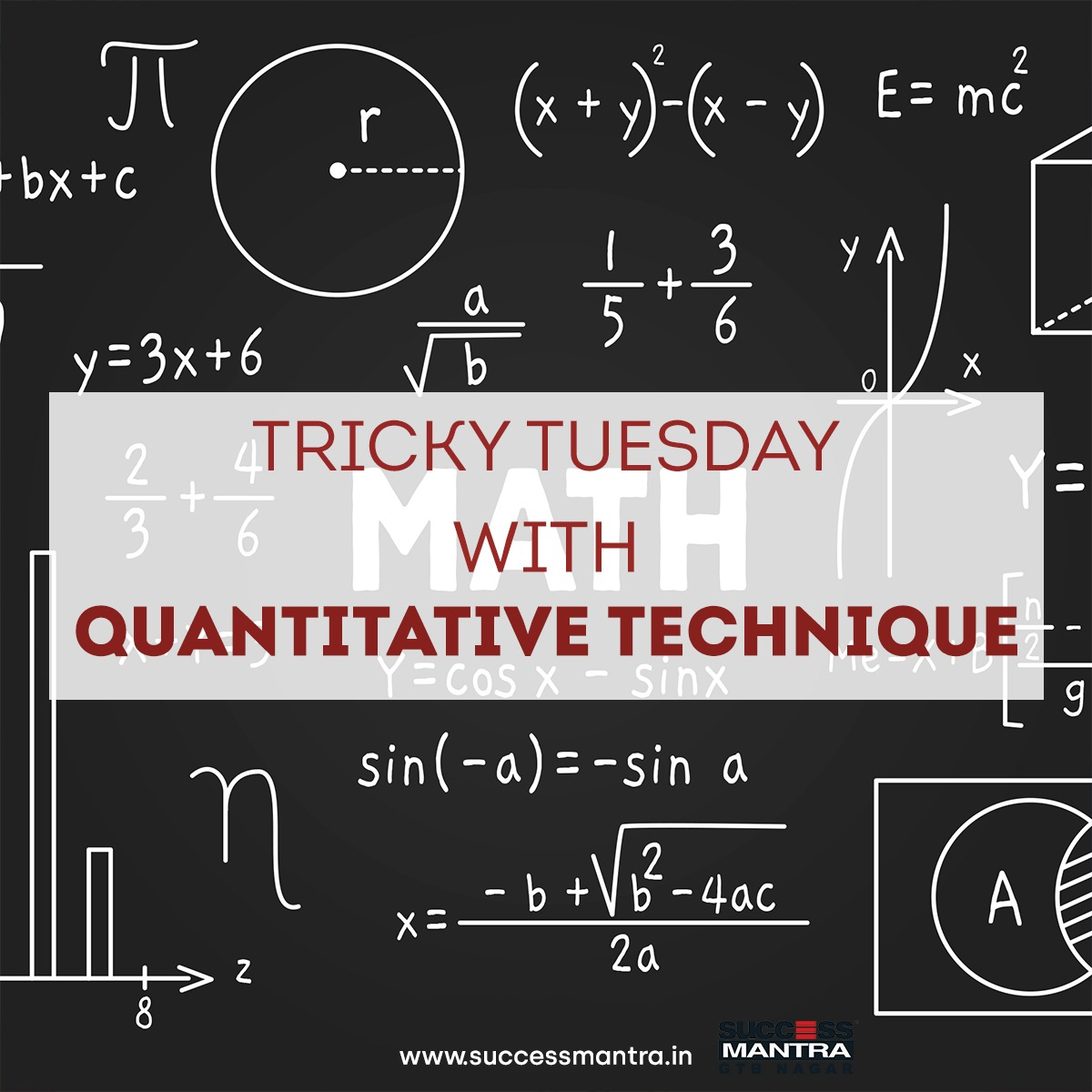## Questions On Quantitative Techniques SMQTQ0221. By selling an article for Rs. 270, a loss of 10 percent is made. Find the C.P. of the article?

A. Rs. 100
B. Rs. 200
C. Rs. 300
D. Rs. 400

2. A dealer marks a T.V. set for 9,000 but agree to gain discount of 20%. Find the selling price of T.V. set.

A. Rs. 1800
B. Rs. 7200
C. Rs. 7800
D. Rs. 6800

3. A sells his goods at 15% discount. Find the price of an article which is sold for Rs. 680.

A. 400
B. 800
C. 1200
D. 1500

4. A trader generally sells goods at 20% discount on marked price. If he wants to make a profit of 25% after allowing a discount of 20%, by what percent should increased price be greater than the original marked price?

A. 15%
B. 65%
C. 25%
D. 20%

5. A shopkeeper allows a discount of 10% on the marked priced of an item but charges a sales tax of 8% on the discounted price. If the customer pays Rs 3,402 as the price including the sales tax, then the marked price is

A. Rs 3,400
B. Rs 3,500
C. Rs 3,600
D. Rs 3,800